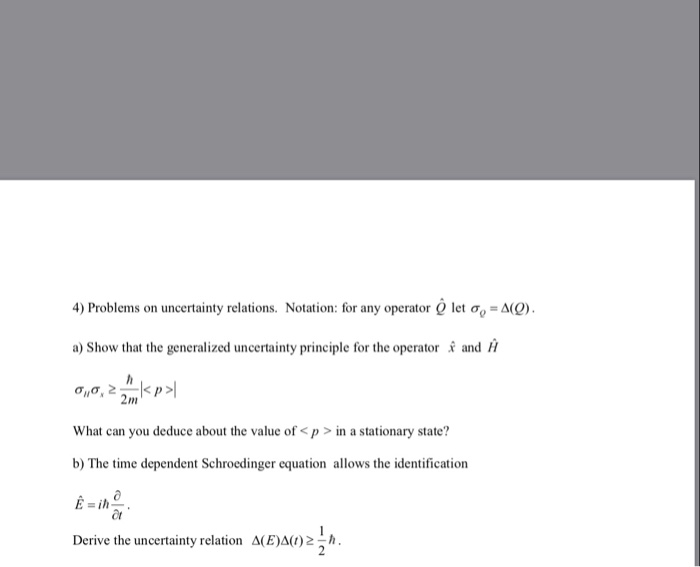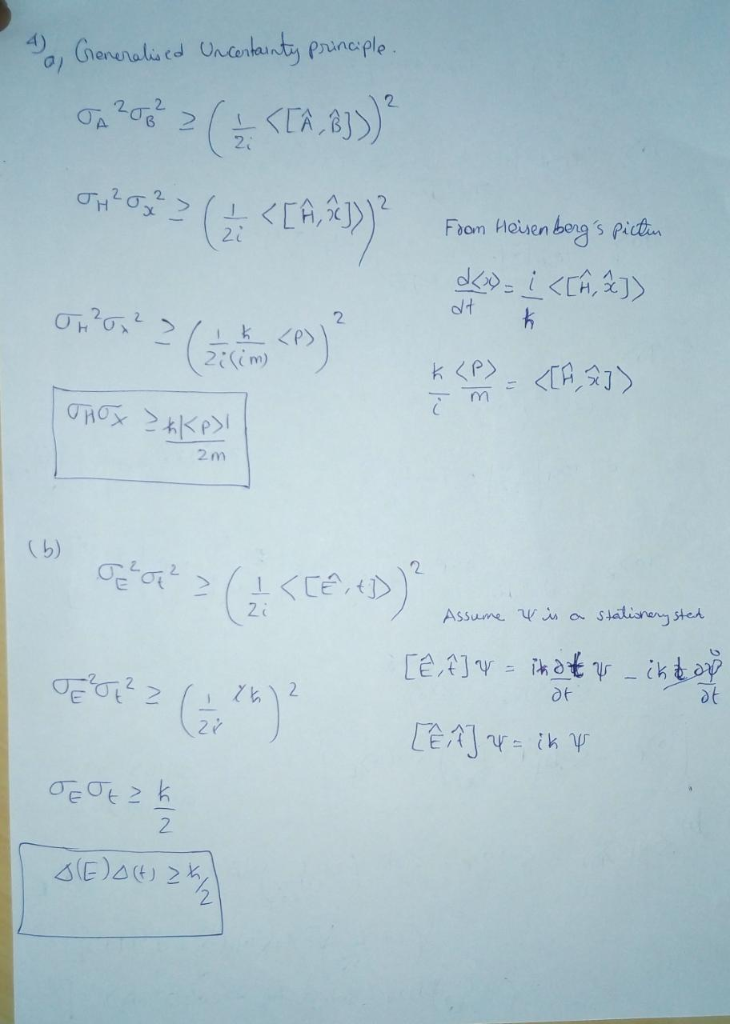# 4) Problems on uncertainty relations. Notation: for any operator O let a-AO) a) Show that the gen...4) Problems on uncertainty relations. Notation: for any operator O let a-AO) a) Show that the generalized uncertainty principle for the operator and H σ//σ,2 |I What can you deduce about the value of in a stationary state? b) The time dependent Schroedinger equation allows the identification E-i Derive the uncertainty relation ACEAO). 2m##### Add Answer of: 4) Problems on uncertainty relations. Notation: for any operator O let a-AO) a) Show that the gen...
More Homework Help Questions Additional questions in this topic.

• #### Let V be a finite-dimensional vector space and let T L(V) be an operator. In this problem you sh...

Need Online Homework Help?